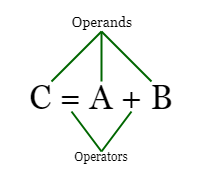## SAP ABAP Operators

The source code of SAP ABAP consists of either statement or comment. Statement is a combination of operators, operands, variables, and keywords.

• Operands : – Operands are the variables which you need to perform particular operation.
• Variables : – Variables is the name given to the memory location.SAP ABAP operators are further classified into different types of operators, they are

1. Arithmetic Operators
2. Comparative Operators
3. Logical Operators.

There should be at least one space between any type of operator. for e.g.

Arithmetic Operators

SAP ABAP/4 supports various arithmetic operators to perform various mathematical functions. For all Arithmetic operators, the default keyword COMPUTE is added at the beginning of the statement.

 Operator Description Example + + (Addition) operator is used to add the values 5+3 = 9 – – (Subtraction) operator is used to subtract the values 5-3  = 9 * * (Multiplication) operator is used to multiply the values 5*3 = 15 ** ** operator is used for exponential of values 5**3 = 125 MOD MOD operator is used for reminder of division of two integer 5 mod 2 / / (Division) is used to divide the values 5/3 = 1.66

Comparative Operators

The different types of comparison abap operators are as follows

 Operator Description Example < or LT Less than A < B or A LT B <= or LE Less than or equal A <= B or A LE B > or GT Greater than A > B or A GT B >= or GE Greater than or equal A >= B or A GE B = or EQ Equal A = B or A = B <> or NE Not Equal A <> B or A NE B

Logical Operators

Logical operators are used to check the results of more than one statement combination.

 Operator Description Example AND AND (Is True) operator is used when all the statements are true IF A > B AND A > C A is Biggest. ENDIF OR OR (Is True) operator is used when at least one statement is true A < B OR A < C NOT NOT ?